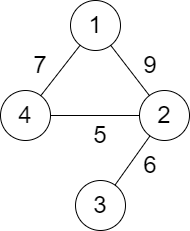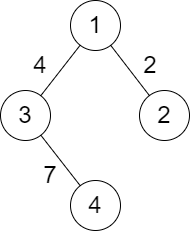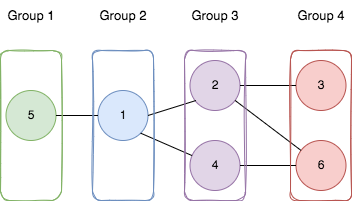# weekly-contest-322

## A

### Statement

• 例如，`"Hello World"``"HELLO"``"hello world hello world"` 都是符合要求的句子。

• 单词的最后一个字符和下一个单词的第一个字符相等。
• 最后一个单词的最后一个字符和第一个单词的第一个字符相等。

``````输入：sentence = "leetcode exercises sound delightful"

- leetcode 的最后一个字符和 exercises 的第一个字符相等。
- exercises 的最后一个字符和 sound 的第一个字符相等。
- sound 的最后一个字符和 delightful 的第一个字符相等。
- delightful 的最后一个字符和 leetcode 的第一个字符相等。

``````输入：sentence = "eetcode"

- eetcode 的最后一个字符和 eetcode 的第一个字符相等。

``````输入：sentence = "Leetcode is cool"

- Leetcode 的最后一个字符和 is 的第一个字符 不 相等。

• `1 <= sentence.length <= 500`
• `sentence` 仅由大小写英文字母和空格组成
• `sentence` 中的单词由单个空格进行分隔
• 不含任何前导或尾随空格

A sentence is a list of words that are separated by a single space with no leading or trailing spaces.

• For example, `"Hello World"`, `"HELLO"`, `"hello world hello world"` are all sentences.

Words consist of only uppercase and lowercase English letters. Uppercase and lowercase English letters are considered different.

A sentence is circular if:

• The last character of a word is equal to the first character of the next word.
• The last character of the last word is equal to the first character of the first word.

For example, `"leetcode exercises sound delightful"`, `"eetcode"`, `"leetcode eats soul" `are all circular sentences. However, `"Leetcode is cool"`, `"happy Leetcode"`, `"Leetcode"` and `"I like Leetcode"` are not circular sentences.

Given a string `sentence`, return `true` if it is circular. Otherwise, return `false`.

Example 1:

``````Input: sentence = "leetcode exercises sound delightful"
Output: true
Explanation: The words in sentence are ["leetcode", "exercises", "sound", "delightful"].
- leetcode's last character is equal to exercises's first character.
- exercises's last character is equal to sound's first character.
- sound's last character is equal to delightful's first character.
- delightful's last character is equal to leetcode's first character.
The sentence is circular.``````

Example 2:

``````Input: sentence = "eetcode"
Output: true
Explanation: The words in sentence are ["eetcode"].
- eetcode's last character is equal to eetcode's first character.
The sentence is circular.``````

Example 3:

``````Input: sentence = "Leetcode is cool"
Output: false
Explanation: The words in sentence are ["Leetcode", "is", "cool"].
- Leetcode's last character is not equal to is's first character.
The sentence is not circular.``````

Constraints:

• `1 <= sentence.length <= 500`
• `sentence` consist of only lowercase and uppercase English letters and spaces.
• The words in `sentence` are separated by a single space.
• There are no leading or trailing spaces.

### Solution

``````#include <bits/stdc++.h>
#include <ext/pb_ds/assoc_container.hpp>
#include <ext/pb_ds/tree_policy.hpp>

#define endl "\n"
#define fi first
#define se second
#define all(x) begin(x), end(x)
#define rall rbegin(a), rend(a)
#define bitcnt(x) (__builtin_popcountll(x))
#define complete_unique(a) a.erase(unique(begin(a), end(a)), end(a))
#define mst(x, a) memset(x, a, sizeof(x))
#define MP make_pair

using ll = long long;
using ull = unsigned long long;
using db = double;
using ld = long double;
using VLL = std::vector<ll>;
using VI = std::vector<int>;
using PII = std::pair<int, int>;
using PLL = std::pair<ll, ll>;

using namespace __gnu_pbds;
using namespace std;
template <typename T>
using ordered_set = tree<T, null_type, less<T>, rb_tree_tag, tree_order_statistics_node_update>;

template <typename T, typename S>
inline bool chmax(T &a, const S &b) {
return a < b ? a = b, 1 : 0;
}

template <typename T, typename S>
inline bool chmin(T &a, const S &b) {
return a > b ? a = b, 1 : 0;
}

#ifdef LOCAL
#include <debug.hpp>
#else
#define dbg(...)
#endif

class Solution {
public:
bool isCircularSentence(string s) {
int n = int(s.size());

for (int i = 1; i < n - 1; i++) {
if (s[i] == ' ') {
if (s[i - 1] != s[i + 1]) {
return false;
}
}
}

return s == s.end()[-1];
}
};

#ifdef LOCAL

int main() {
return 0;
}

#endif
``````

## B

### Statement

``````输入：skill = [3,2,5,1,3,4]

``````

``````输入：skill = [3,4]

``````

``````输入：skill = [1,1,2,3]

``````

• `2 <= skill.length <= 105`
• `skill.length` 是偶数
• `1 <= skill[i] <= 1000`

You are given a positive integer array `skill` of even length `n` where `skill[i]` denotes the skill of the `ith` player. Divide the players into `n / 2` teams of size `2` such that the total skill of each team is equal.

The chemistry of a team is equal to the product of the skills of the players on that team.

Return the sum of the chemistry of all the teams, or return `-1` if there is no way to divide the players into teams such that the total skill of each team is equal.

Example 1:

``````Input: skill = [3,2,5,1,3,4]
Output: 22
Explanation:
Divide the players into the following teams: (1, 5), (2, 4), (3, 3), where each team has a total skill of 6.
The sum of the chemistry of all the teams is: 1 * 5 + 2 * 4 + 3 * 3 = 5 + 8 + 9 = 22.
``````

Example 2:

``````Input: skill = [3,4]
Output: 12
Explanation:
The two players form a team with a total skill of 7.
The chemistry of the team is 3 * 4 = 12.
``````

Example 3:

``````Input: skill = [1,1,2,3]
Output: -1
Explanation:
There is no way to divide the players into teams such that the total skill of each team is equal.
``````

Constraints:

• `2 <= skill.length <= 105`
• `skill.length` is even.
• `1 <= skill[i] <= 1000`

### Solution

``````#include <bits/stdc++.h>
#include <ext/pb_ds/assoc_container.hpp>
#include <ext/pb_ds/tree_policy.hpp>

#define endl "\n"
#define fi first
#define se second
#define all(x) begin(x), end(x)
#define rall rbegin(a), rend(a)
#define bitcnt(x) (__builtin_popcountll(x))
#define complete_unique(a) a.erase(unique(begin(a), end(a)), end(a))
#define mst(x, a) memset(x, a, sizeof(x))
#define MP make_pair

using ll = long long;
using ull = unsigned long long;
using db = double;
using ld = long double;
using VLL = std::vector<ll>;
using VI = std::vector<int>;
using PII = std::pair<int, int>;
using PLL = std::pair<ll, ll>;

using namespace __gnu_pbds;
using namespace std;
template <typename T>
using ordered_set = tree<T, null_type, less<T>, rb_tree_tag, tree_order_statistics_node_update>;

template <typename T, typename S>
inline bool chmax(T &a, const S &b) {
return a < b ? a = b, 1 : 0;
}

template <typename T, typename S>
inline bool chmin(T &a, const S &b) {
return a > b ? a = b, 1 : 0;
}

#ifdef LOCAL
#include <debug.hpp>
#else
#define dbg(...)
#endif

class Solution {
public:
long long dividePlayers(vector<int> &skill) {
int sum = accumulate(all(skill), 0);

int n = int(skill.size());
int m = n / 2;

int need = sum / m;

sort(all(skill));

ll res = 0;

int r = n - 1;
for (int i = 0; i < r; i++, r--) {
if (skill[i] + skill[r] != need) {
return -1;
}

res += 1ll * skill[i] * skill[r];
}

return res;
}
};

#ifdef LOCAL

int main() {
return 0;
}

#endif
``````

## C

### Statement

• 一条路径指的是两个城市之间的道路序列。
• 一条路径可以 多次 包含同一条道路，你也可以沿着路径多次到达城市 `1` 和城市 `n` 。
• 测试数据保证城市 `1` 和城市`n` 之间 至少 有一条路径。``````输入：n = 4, roads = [[1,2,9],[2,3,6],[2,4,5],[1,4,7]]

````````````输入：n = 4, roads = [[1,2,2],[1,3,4],[3,4,7]]

``````

• `2 <= n <= 105`
• `1 <= roads.length <= 105`
• `roads[i].length == 3`
• `1 <= ai, bi <= n`
• `ai != bi`
• `1 <= distancei <= 104`
• 不会有重复的边。
• 城市 `1` 和城市 `n` 之间至少有一条路径。

You are given a positive integer `n` representing `n` cities numbered from `1` to `n`. You are also given a 2D array `roads` where `roads[i] = [ai, bi, distancei]` indicates that there is a bidirectional road between cities `ai` and `bi` with a distance equal to `distancei`. The cities graph is not necessarily connected.

The score of a path between two cities is defined as the minimum distance of a road in this path.

Return the minimum possible score of a path between cities `1` and `n`.

Note:

• A path is a sequence of roads between two cities.
• It is allowed for a path to contain the same road multiple times, and you can visit cities `1` and `n` multiple times along the path.
• The test cases are generated such that there is at least one path between `1` and `n`.

Example 1:``````Input: n = 4, roads = [[1,2,9],[2,3,6],[2,4,5],[1,4,7]]
Output: 5
Explanation: The path from city 1 to 4 with the minimum score is: 1 -> 2 -> 4. The score of this path is min(9,5) = 5.
It can be shown that no other path has less score.
``````

Example 2:``````Input: n = 4, roads = [[1,2,2],[1,3,4],[3,4,7]]
Output: 2
Explanation: The path from city 1 to 4 with the minimum score is: 1 -> 2 -> 1 -> 3 -> 4. The score of this path is min(2,2,4,7) = 2.
``````

Constraints:

• `2 <= n <= 105`
• `1 <= roads.length <= 105`
• `roads[i].length == 3`
• `1 <= ai, bi <= n`
• `ai != bi`
• `1 <= distancei <= 104`
• There are no repeated edges.
• There is at least one path between `1` and `n`.

### Solution

``````#include <bits/stdc++.h>
#include <ext/pb_ds/assoc_container.hpp>
#include <ext/pb_ds/tree_policy.hpp>

#define endl "\n"
#define fi first
#define se second
#define all(x) begin(x), end(x)
#define rall rbegin(a), rend(a)
#define bitcnt(x) (__builtin_popcountll(x))
#define complete_unique(a) a.erase(unique(begin(a), end(a)), end(a))
#define mst(x, a) memset(x, a, sizeof(x))
#define MP make_pair

using ll = long long;
using ull = unsigned long long;
using db = double;
using ld = long double;
using VLL = std::vector<ll>;
using VI = std::vector<int>;
using PII = std::pair<int, int>;
using PLL = std::pair<ll, ll>;

using namespace __gnu_pbds;
using namespace std;
template <typename T>
using ordered_set = tree<T, null_type, less<T>, rb_tree_tag, tree_order_statistics_node_update>;

template <typename T, typename S>
inline bool chmax(T &a, const S &b) {
return a < b ? a = b, 1 : 0;
}

template <typename T, typename S>
inline bool chmin(T &a, const S &b) {
return a > b ? a = b, 1 : 0;
}

#ifdef LOCAL
#include <debug.hpp>
#else
#define dbg(...)
#endif

const int INF = 0x3f3f3f3f;

class Solution {
public:
vector<vector<tuple<int, int>>> g{};
int n;

int bfs() {
int st = 1;
auto dis = vector<int>(n + 5, INF);

queue<int> q;
q.push(st);

while (!q.empty()) {
int u = q.front();
q.pop();

for (const auto &[v, d] : g[u]) {
int cur_dis = min(dis[u], d);
if (cur_dis < dis[v]) {
dis[v] = cur_dis;
q.push(v);
}
}
}

return dis[n];
}

int minScore(int n, vector<vector<int>> &roads) {
g.clear();
g = vector<vector<tuple<int, int>>>(n + 5, vector<tuple<int, int>>());
this->n = n;

for (const auto &r : roads) {
int u = r;
int v = r;
int d = r;

g[u].push_back(make_tuple(v, d));
g[v].push_back(make_tuple(u, d));
}

return bfs();
}
};

#ifdef LOCAL

int main() {
return 0;
}

#endif
``````

## D

### Statement

• 图中每个节点都只属于一个组。
• 图中每条边连接的两个点 `[ai, bi]` ，如果 `ai` 属于编号为 `x` 的组，`bi` 属于编号为 `y` 的组，那么 `|y - x| = 1` 。``````输入：n = 6, edges = [[1,2],[1,4],[1,5],[2,6],[2,3],[4,6]]

- 节点 5 在第一个组。
- 节点 1 在第二个组。
- 节点 2 和节点 4 在第三个组。
- 节点 3 和节点 6 在第四个组。

``````

``````输入：n = 3, edges = [[1,2],[2,3],[3,1]]

``````

• `1 <= n <= 500`
• `1 <= edges.length <= 104`
• `edges[i].length == 2`
• `1 <= ai, bi <= n`
• `ai != bi`
• 两个点之间至多只有一条边。

You are given a positive integer `n` representing the number of nodes in an undirected graph. The nodes are labeled from `1` to `n`.

You are also given a 2D integer array `edges`, where `edges[i] = [ai, bi]` indicates that there is a bidirectional edge between nodes `ai` and `bi`. Notice that the given graph may be disconnected.

Divide the nodes of the graph into `m` groups (1-indexed) such that:

• Each node in the graph belongs to exactly one group.
• For every pair of nodes in the graph that are connected by an edge `[ai, bi]`, if `ai` belongs to the group with index `x`, and `bi` belongs to the group with index `y`, then `|y - x| = 1`.

Return the maximum number of groups (i.e., maximum `m`) into which you can divide the nodes. Return `-1` if it is impossible to group the nodes with the given conditions.

Example 1:``````Input: n = 6, edges = [[1,2],[1,4],[1,5],[2,6],[2,3],[4,6]]
Output: 4
Explanation: As shown in the image we:
- Add node 5 to the first group.
- Add node 1 to the second group.
- Add nodes 2 and 4 to the third group.
- Add nodes 3 and 6 to the fourth group.
We can see that every edge is satisfied.
It can be shown that that if we create a fifth group and move any node from the third or fourth group to it, at least on of the edges will not be satisfied.
``````

Example 2:

``````Input: n = 3, edges = [[1,2],[2,3],[3,1]]
Output: -1
Explanation: If we add node 1 to the first group, node 2 to the second group, and node 3 to the third group to satisfy the first two edges, we can see that the third edge will not be satisfied.
It can be shown that no grouping is possible.
``````

Constraints:

• `1 <= n <= 500`
• `1 <= edges.length <= 104`
• `edges[i].length == 2`
• `1 <= ai, bi <= n`
• `ai != bi`
• There is at most one edge between any pair of vertices.

### Solution

``````#include <bits/stdc++.h>
#include <ext/pb_ds/assoc_container.hpp>
#include <ext/pb_ds/tree_policy.hpp>

#define endl "\n"
#define fi first
#define se second
#define all(x) begin(x), end(x)
#define rall rbegin(a), rend(a)
#define bitcnt(x) (__builtin_popcountll(x))
#define complete_unique(a) a.erase(unique(begin(a), end(a)), end(a))
#define mst(x, a) memset(x, a, sizeof(x))
#define MP make_pair

using ll = long long;
using ull = unsigned long long;
using db = double;
using ld = long double;
using VLL = std::vector<ll>;
using VI = std::vector<int>;
using PII = std::pair<int, int>;
using PLL = std::pair<ll, ll>;

using namespace __gnu_pbds;
using namespace std;
template <typename T>
using ordered_set = tree<T, null_type, less<T>, rb_tree_tag, tree_order_statistics_node_update>;

template <typename T, typename S>
inline bool chmax(T &a, const S &b) {
return a < b ? a = b, 1 : 0;
}

template <typename T, typename S>
inline bool chmin(T &a, const S &b) {
return a > b ? a = b, 1 : 0;
}

#ifdef LOCAL
#include <debug.hpp>
#else
#define dbg(...)
#endif

class Solution {
public:
vector<int> color, node;
vector<vector<int>> G;
int n;

bool dfs(int u, int col) {
node.push_back(u);
color[u] = col;
for (const auto &v : G[u]) {
if (color[v] != -1) {
if (color[v] == col) {
return false;
}

continue;
}

if (!dfs(v, col ^ 1)) {
return false;
}
}

return true;
}

int bfs(int st) {
queue<int> q;
q.push(st);

auto dep = vector<int>(n + 5, 0);
dep[st] = 1;

int mx_dep = 1;

while (!q.empty()) {
int u = q.front();
q.pop();

for (const auto &v : G[u]) {
if (dep[v] == 0) {
dep[v] = dep[u] + 1;
mx_dep = max(mx_dep, dep[v]);
q.push(v);
}
}
}

return mx_dep;
}

int magnificentSets(int n, vector<vector<int>> &edges) {
this->n = n;

G = vector<vector<int>>(n + 5, vector<int>());

for (const auto &e : edges) {
int u = e;
int v = e;
G[u].push_back(v);
G[v].push_back(u);
}

int res = 0;

color = vector<int>(n + 5, -1);

for (int i = 1; i <= n; i++) {
node.clear();
if (color[i] == -1) {
if (!dfs(i, 0)) {
return -1;
}

int cur_res = 0;

for (const auto &a : node) {
cur_res = max(cur_res, bfs(a));
}

res += cur_res;
}
}

return res;
}
};

#ifdef LOCAL

int main() {
return 0;
}

#endif
``````